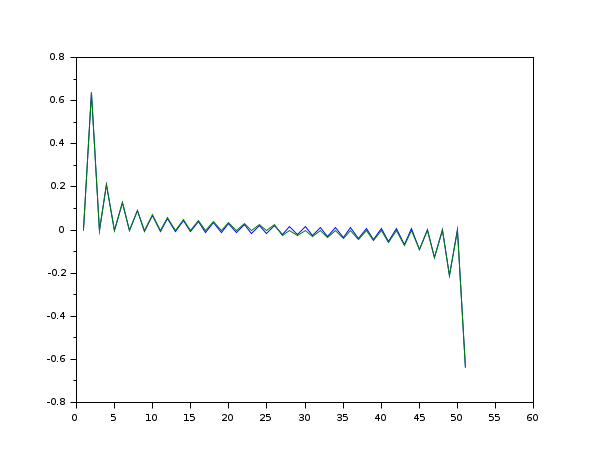Scilab Home page | Wiki | Bug tracker | Forge | Mailing list archives | ATOMS | File exchange
Change language to: Français - Português - 日本語 - Русский
Scilab Help >> Signal Processing > Filters > hilbert

hilbert

Discrete-time analytic signal computation of a real signal using Hilbert transform

x=hilbert(xr)

Arguments

xr

real vector : the real signal samples

x

Complex vector: the discrete-time analytic signal.

Description

Returns theanalytic signal, from a real data sequence.

The analytic signal x= xr + i*xi has a real part, xr, which is the original data, and an imaginary part, xi, which contains the Hilbert transform. The imaginary part is a version of the original real sequence with a 90° phase shift.

References

http://ieeexplore.ieee.org/iel5/78/16975/00782222.pdf?arnumber=782222

Marple, S.L., "Computing the discrete-time analytic signal via FFT," IEEE Transactions on Signal Processing, Vol. 47, No.9 (September 1999), pp.2600-2603

• window — compute symmetric window of various type
• hil — FIR approximation to a Hilbert transform filter

Examples

//compare the discrete-time analytic signal imaginary part of the impulse real signal
// with the FIR approximation of the Hilbert transform filter
m=25;
n=2*m+1;
y=hilbert(eye(n,1));
h=hilb(n)';
h=[h((m+1):\$);h(1:m)];
plot([imag(y) h])